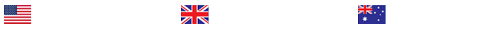Explain how the standard deviation (SD) and the deviation of a single score differ in the information they provide.

Application: Measures of Central Tendency and Variability

While researchers typically show frequency distributions in a table as a way to organize large amounts of data, you may have also seen frequency distributions displayed graphically, using histograms or smooth curves. Consider the bell curve, or normal distribution, and remember the first time you saw one. What can the frequency of occurrence tell us about a characteristic or phenomenon? Can it tell you how much better or worse you performed on a test when compared to the scores of your classmates? Imagine that you collected those test scores and created a frequency distribution. There might be, for example, many scores clustered around the 90% mark and no scores in the 0% to 60% range. Refer to the following graphic for more information. If your frequency histogram only included the range of observed scores (60% to 100%) instead of the entire range of possible scores (0% to 100%), it might appear that you had a normal distribution when really there was a negative skew. What other factors can you consider when planning how to interpret data?

Frequency distributions, particularly the normal curve, are an ongoing concept in this class. The normal distribution is very common in behavioral research. When you describe data with many different scores, you will understand more about them if you know the resulting distribution type. For example, if you notice a test score frequency distribution in the shape of two equal humps, or in a bimodal distribution, you can say with some certainty that scores fell into two ranges showing two discrete groupings of students’ scores. This Assignment focuses on your ability to understand and distinguish among the different types of frequency distributions, measures of central tendency, and measures of variability and understand what they mean in relation to a sample.

To prepare for this Assignment, review mean, median, and mode as well as the different types of distributions in your textbook.

Note: As you move forward through the course, you will see terms that you focused on in previous chapters. Each time you practice, you should know how to state hypotheses and be able to identify your independent and dependent variables. Each Assignment will contribute to your depth of knowledge so that you can understand more and more about conducting research studies.

Scenario:

Recall that for this week’s Discussion you considered data related to opening or attracting a new restaurant. Now consider that you ask 20 participants to estimate how many times a month they go out to dinner and you receive these responses:

1, 2, 5, 8, 2, 4, 8, 4, 2, 3, 6, 8, 7, 5, 8, 4, 0, 7, 6, and 18.

Assignment:

To complete this Assignment, submit by Day 7 calculations of the following measures of central tendency and variability using the data set provided. Include an explanation of how you calculated each measure and what information each measure gives you about the dining behavior of the sample. Finally, create a data file in SPSS and run analyses to find the mean and standard deviation. Note: Your hand-calculated mean and standard deviation will differ somewhat from the calculations in SPSS due to rounding.

Hand-calculated mean:

SPSS mean:

Median:

Mode:

Range:

Deviation of the highest score from the mean:

Hand-calculated standard deviation (Please also state the hand-calculated values for ∑X2and(∑X)2.):

SPSS standard deviation:

Explain how the standard deviation (SD) and the deviation of a single score differ in the information they provide. Explain how each measure (mean, median, mode, deviation of the highest score from the mean, and standard deviation) would change if the score of 18 was eliminated from the data set.

Explain the type of distribution (positive skew, negative skew, bimodal distribution, or normal distribution) your data create. Explain how you know the type of distribution and what the data tells you about your sample.

### Last Completed Projects

# topic title discipline academic level pages delivered
6
Writer's choice
University
2
1 hour 32 min
7
Wise Approach to
Philosophy
College
2
2 hours 19 min
8
1980's and 1990
History
College
3
2 hours 20 min
9
pick the best topic
Finance
School
2
2 hours 27 min
10
finance for leisure
Finance
University
12
2 hours 36 min